# Rotating Magnetic Field in Three-Phase Induction Motor Notes | Study Basic Electrical Technology - Electrical Engineering (EE)

## Electrical Engineering (EE): Rotating Magnetic Field in Three-Phase Induction Motor Notes | Study Basic Electrical Technology - Electrical Engineering (EE)

The document Rotating Magnetic Field in Three-Phase Induction Motor Notes | Study Basic Electrical Technology - Electrical Engineering (EE) is a part of the Electrical Engineering (EE) Course Basic Electrical Technology.
All you need of Electrical Engineering (EE) at this link: Electrical Engineering (EE)

Rotating Magnetic Field in Three-phase Induction Motor

In the previous module, containing six lessons (23-28), mainly, the study of the singlephase two-winding Transformers – a static machine, fed from ac supply, has been presented. In this module, containing six lessons (29-34), mainly, the study of Induction motors, fed from balanced three-phase supply, will be described.

In this (first) lesson of this module, the formation of rotating magnetic field in the air gap of an induction motor, is described, when the three-phase balanced winding of the stator is supplied with three-phase balanced voltage. The balanced winding is of the same type, as given in lesson no. 18, for a three-phase ac generator.

Keywords:  Induction motor, rotating magnetic field, three-phase balanced winding, and balanced voltage.

After going through this lesson, the students will be able to answer the following questions:

1. How a rotating magnetic field is formed in the air gap of a three-phase Induction motor, when the balanced winding of the stator is fed from a balanced supply?
2. Why does the magnitude of the magnetic field remain constant, and also what is the speed of rotation of the magnetic field, so formed? Also what is meant by the term ‘synchronous speed’?

Three-phase Induction Motor

A three-phase balanced winding in the stator of the Induction motor (IM) is shown in Fig. 29.1 (schematic form). In a three-phase balanced winding, the number of turns in three windings, is equal, with the angle between the adjacent phases, say R & Y, is (electrical). Same angle of  (elec.) is also between the phases, Y & B.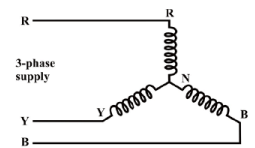Fig. 29.1: Schematic diagram of the stator windings in a three-phase induction motor.

A three-phase balanced voltage, with the phase sequence as R-Y-B, is applied to the above winding. In a balanced voltage, the magnitude of the voltage in each phase, assumed to be in star in this case, is equal, with the phase angle of the voltage between the adjacent phases, say R & Y, being120°.

Rotating Magnetic Field

The three phases of the stator winding (balanced) carry balanced alternating (sinusoidal) currents as shown in Fig. 29.2.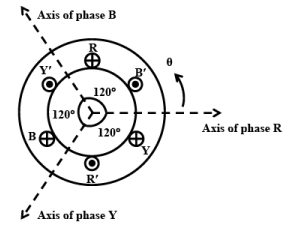Fig. 29.2: The relative location of the magnetic axis of three phases.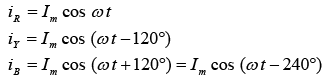Please note that the phase sequence is R-Y-B. Im is the maximum value of the phase currents, and, as the phase currents are balanced, the rms values are equal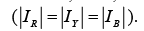Three pulsating mmf waves are now set up in the air-gap, which have a time phase difference of  from each other. These mmf’s are oriented in space along the magnetic axes of the phases, R, Y & B, as illustrated by the concentrated coils in Fig. 29.2. Please note that 120° 2-pole stator is shown, with the angle between the adjacent phases, R & Y as , in both mechanical and electrical terms. Since the magnetic axes are located  apart in space from each other, the three mmf’s are expresses mathematically as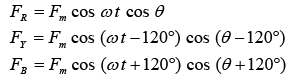wherein it has been considered that the three mmf waves differ progressively in time phase by 120° , i.e. 2π / 3 rad (elect.), and are separated in space phase by 120° , i.e. 2π / 3 rad (elect.). The resultant mmf wave, which is the sum of three pulsating mmf waves, is  F = FR+ FY + FB Substituting the values,

F (θ , t )

= Fm [cos ωt cos θ + cos (ωt − 120°) cos (θ − 120°) + cos (ωt + 120°) cos (θ + 120°)]

The first term of this expression is

cos ωt cos θ = 0.5 [cos (θ − ωt ) + cos (θ + ωt )]

The second term is

cos (ωt − 120°) cos (θ − 120°) = 0.5 [cos (θ − ωt ) + cos (θ + ωt − 240°)]

Similarly, the third term can be rewritten in the form shown.  The expression is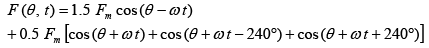Note that

cos (θ + ωt − 240°) = cos (θ + ωt + 120°) , and

cos (θ + ωt + 240°) = cos (θ + ωt − 120°) .

If these two terms are added, then

cos (θ + ωt + 120°) + cos (θ + ωt − 120°) = − cos (θ + ωt )

So, in the earlier expression, the second part of RHS within the capital bracket is zero. In other words, this part represents three unit phasors with a progressive phase difference of 120° , and therefore add up to zero. Hence, the resultant mmf is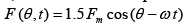So, the resultant mmf is distributed in both space and time. It can be termed as a rotating magnetic field with sinusoidal space distribution, whose space phase angle changes linearly with time as ωt . It therefore rotates at a constant angular speed of ω rad (elect.)/s. This angular speed is called synchronous angular speed (ω s ).

The peak value of the resultant mmf is F peak = 1.5 Fm . The value of  depends on No. of turns/phase, winding current, No. of poles, and winding factor. At m F
ωt = 0 , i.e. when the current in R phase has maximum positive value, F (θ , 0) = 1.5 Fm cos θ , i.e. the mmf wave has its peak value (at θ = 0 ) lying on the axis of R phase, when it carries maximum positive current. At ωt = 2π / 3 (120°) , the phase Y (assumed lagging) has its positive current maximum, so that the peak of the rotating magnetic field (mmf) lying on the axis of Y phase. By the same argument, the peak of the mmf coincides with the axis of phase B at ωt = 4π / 3 (240°) . It is, therefore, seen that the resultant mmf moves from the axis of the leading phase to that of the lagging phase, i.e. from phase R towards phase Y, and then phase B, when the phase sequence of the currents is R-Y-B (R leads Y, and Y leads B). As described in brief later, the direction of rotation of the resultant mmf is reversed by simply changing the phase sequence of currents.

From the above discussion, the following may be concluded: Whenever a balanced three-phase winding with phases distributed in space so that the relative space angle between them is 2π / 3 rad (elect.) [120°] , is fed with balanced three-phase currents with relative phase difference of 2π / 3 rad (elect.) [120°] , the resultant mmf rotates in the airgap at an angular speed of  ωs =2π(f/p) ,  where f is the frequency (Hz) of currents and is No. of pairs of poles for which the winding is designed. The synchronous speed in rpm (r/min) is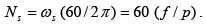The direction of rotation of the mmf is from the leading phase axis to lagging phase axis. This is also valid for q-phase balanced winding, one value of which may be q = 2 (two). For a 2-phase balanced winding, the time and phase angles are (π / 2) rad or 90° (elect.).

Alternatively, this production of rotating magnetic field can be shown by the procedure described. As stated earlier, the input voltage to three-phase balanced winding of the stator is a balanced one with the phase sequence (R-Y-B). This is shown in the sinusoidal voltage waveforms of the three phases, R, Y & B (Fig. 29.3).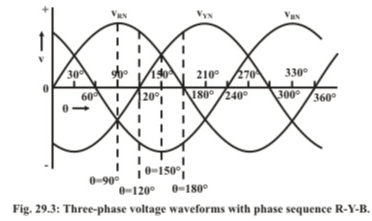A two-pole, three-phase balanced winding in the stator of IM is shown in Fig. 29.4(i)- (a-d), where the winding of each phase, say for example, ( R − R ′ ) is assumed to be concentrated in one slot each, both for forward and return conductors, with required no. as needed. Same is the case for other two phases. Please note that the angle of 1200 is same in both mechanical (as shown) and also electrical terms, as no. of poles is two only. The two (forward and return) parts of the winding in each phase, say R are referred as R and R′ respectively. So, also for two other phases, Y & B as shown.

Let us first consider, what happens at the time instant tof the voltage waveforms as given in Fig. 29.3. At this instant, the voltage in the R-phase is positive maximum (θ1 = 90° ), while the two other voltages in the phases, Y & B are half of the maximum value, and also negative. The three waveforms are represented by the following equations: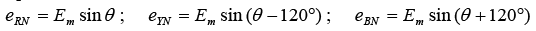where,

f = Supply frequency (Hz or c/s)

ω = 2π f = Angular frequency (rad/s)

E m= Maximum value of the voltage, or induced emf in each phase The currents in three windings are shown in Fig. 29.4(a)-(i). For (θ1 = 90° ), the current in R-phase in positive maximum, while the currents in both Y and B phases are negative, with magnitude as half of maximum value (0.5). The fluxes due to the currents in the windings are shown in Fig. 29.4(a)-(ii). It may be noted that ΦR is taken as reference,  while  Φleads ΦR by  and Φlags Φ R by 60,as can be observed from the direction of currents in all three phases as given earlier. If the fluxes are added to find the resultant in phasor form, the magnitude is found as (1.5.Φ ) It may be noted that this magnitude is same as that found mathematically earlier. Its direction is also shown in same figure. The resultant flux is given by,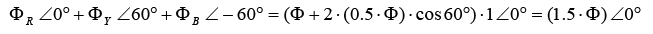Now, let us shift to the instant, t2 2 = 120° ) as shown in Fig. 29.3. The voltages in the two phases, R & B are ( √3 / 2 = 0.866 ) times the maximum value, with R-phase as positive and B-phase as negative. The voltage in phase Y is zero. This is shown in Fig. 29.4(b)-(i). The fluxes due to the currents in the windings are shown in Fig. 29.4(b)-(ii). As given earlier,ΦR is taken as reference here, while  Φ B lags  Φ R by 600. Please note the direction of the currents in both R and B phases. If the fluxes are added to find the resultant in phasor form, the magnitude is found as (1.5 .Φ ) the same figure. The resultant flux is given by,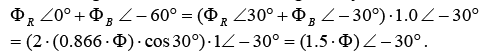It may be noted that the magnitude of the resultant flux remains constant, with its direction shifting by 30° = 120° − 90° in the clockwise direction from the previous instant.

Similarly, if we now shift to the instant, t33 = 150° ) as shown in Fig. 29.3. The voltage in the B-phase is negative maximum, while the two other voltages in the phases, R & Y, are half of the maximum value (0.5), and also positive. This is shown in Fig. 29.4(c)-(i). The fluxes due to the currents in the windings are shown in Fig. 29.4(c)-(ii). The reference of flux direction ( ΦR ) is given earlier, and is not repeated here. If the fluxes are added to find the resultant in phasor form, the magnitude is found as (1.5 ⋅ Φ ). The direction is shown in the same figure. The resultant flux is given by,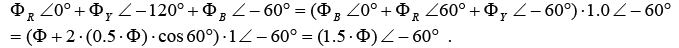It may be noted that the magnitude of the resultant flux remains constant, with the direction shifting by another 30° in the clockwise direction from the previous instant.   If we now consider the instant, t44 = 180° ) as shown in Fig. 29.3. The voltages in the two phases, Y & B are ( √3 / 2 = 0.866) times the maximum value, with Y-phase as positive and B-phase as negative. The voltage in phase R is zero. This is shown in Fig. 29.4(d)-(i). The fluxes due to the currents in the windings are shown in Fig. 29.4(d)-(ii).If the fluxes are added to find the resultant in phasor form, the magnitude is found as ( 1.5 ⋅ Φ ). The direction is shown in the same figure. The resultant flux is given by,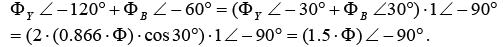It may be noted that the magnitude of the resultant flux remains constant, with its direction shifting by another 30° in the clockwise direction from the previous instant.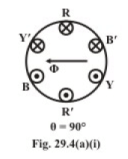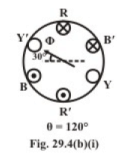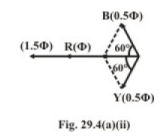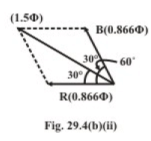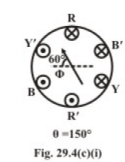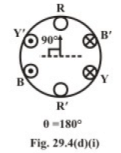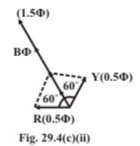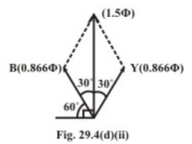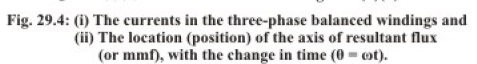A study of the above shows that, as we move from t11 = 90° ) to t44 = 180° ) along the voltage waveforms (Fig. 29.3), the magnitude of the resultant flux remains constant at the value ( 1.5 ⋅ Φ) as shown in Fig. 29.4(ii)-(a-d). If another point, say t55=2100) is taken, it can be easily shown that the magnitude of the resultant flux at that instant remains same at (1.5 ⋅ Φ ), which value is obtained mathematically earlier. If any other arbitrary point, t (θ ) on the waveform is taken, the magnitude of the resultant flux at that instant remains same at (1.5 ⋅ Φ ). Also, it is seen that the axis of the resultant flux moves through 90° , as the angle, θ changes from  to , i.e. by the same angle of . So, if we move through one cycle of the waveform, by  (electrical), the axis of the resultant flux also moves through  (2-pole stator), i.e. one complete revolution. The rotating magnetic field moves in the clockwise direction as shown, from phase R to phase Y. Please note that, for 2-pole configuration as in this case, the mechanical and electrical angles are same. So, the speed of the rotating magnetic field for this case is 50 rev/sec (rps), or 3,000 rev/min (rpm), as the supply frequency is 50 Hz or c/s, with its magnitude, i.e. resultant, remaining same.

Four-Pole Stator

A 4-pole stator with balanced three-phase winding (Fig. 29.5) is taken as an example. The winding of each phase (one part only), say for example, ( R1 − R1′ ) is assumed to be concentrated in one slot each, both for forward and return conductors, with required no. as needed. Same is the case for other two phases. The connection of two parts of the winding in R-phase, is also shown in the same figure. The windings for each of three phases are in two parts, with the mechanical angle between the start of adjacent windings being  600 only, whereas the electrical angle remaining same at 1200 As two pairs of poles are there, electrically two cycles, i.e. 720are there for one complete revolution,with each N-S pair for one cycle of 3600 but the mechanical angle is only  3600  If we move through one cycle of the waveform, by 3600 (electrical), the axis of the resultant flux in this case moves through a mechanical angle of 180 i.e. one pole pair (3600- elec.), or half revolution only. As stated earlier, for the resultant flux axis to make one complete revolution (3600- mech.), two cycles of the waveform (7200- elec.), are required, as No. of poles ( ) is four (4). So, for the supply frequency of  50 Hz (c/s), the speed of the rotating magnetic field is given by,

n s = (2 ⋅ f ) / p = f /( p / 2) = 50 /(4 / 2) = 25 rev/sec (rps), or N s = 1,500 rev/min (rpm).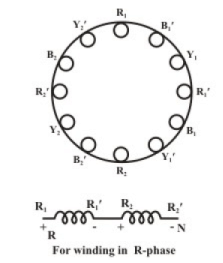Fig- 29.5: Three-phase balanced ™indiums in a 4-pole stator.

The relation between the synchronous speed, i.e. the speed of the rotating magnetic field, in rpm and the supply frequency in Hz, is given by

N = (60 × 2 ⋅ f ) / p = (120 ⋅ f ) / p

To take another example of a 6-pole stator, in which, for 50 Hz supply, the synchronous speed is 1,000 rpm, obtained by using the above formula.

The Reversal of Direction of Rotating Magnetic Field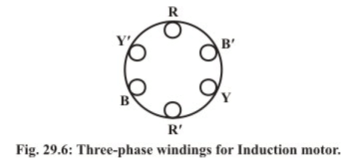The direction of the rotating magnetic field is reversed by changing the phase sequence to R-B-Y, i.e. changing only the connection of any two of the three phases, and keeping the third one same. The schematic of the balanced three-phase winding for a 2-pole stator, with the winding of each phase assumed to be concentrated in one slot, is redrawn in Fig. 29.6, which is same as shown in Fig. 29.4(i) (a-d). The space phase between the adjacent windings of any two phases (say R & Y, or R & B) is 120° , i.e. 2π / 3 rad (elect.), as a 2-pole stator is assumed. Also, it may be noted that, while the connection to phase R remains same, but the phases, Y and B of the winding are now connected to the phases, B and Y of the supply respectively. The waveforms for the above phase sequence (R-B-Y) are shown in Fig. 29.7. Please note that, the voltage in phase R leads the voltage in phase B, and the voltage in phase B leads the voltage in phase Y. As compared to the three waveforms shown in Fig. 29.3, the two waveforms of the phases Y & B change, while the reference phase R remains same, with the phase sequence reversed as given earlier. The currents in three phases of the stator winding are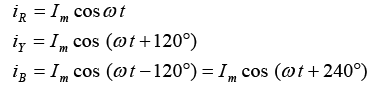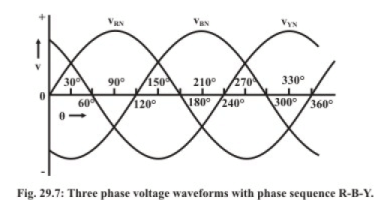Without going into the details of the derivation, which has been presented in detail earlier, the resultant mmf wave is obtained as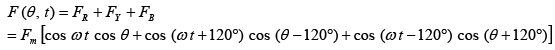As shown earlier, the first term of this expression is

cos ωt cos θ = 0.5 [cos (θ + ωt ) + cos (θ − ωt )]

The second term is

cos (ωt + 120°) cos (θ − 120°) = 0.5 [cos (θ + ωt ) + cos (θ − ωt − 240°)]

Similarly, the third term can be rewritten in the form shown.  The expression is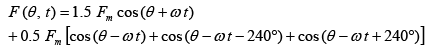As shown or derived earlier, the expression, after simplification, is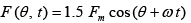Note that the second part of the expression within square bracket is zero.  It can be shown that the rotating magnetic field now moves in the reverse (i.e., anticlockwise) direction (Fig. 29.6), from phase R to phase B (lagging phase R by 1200) which is the reverse of earlier (clockwise) direction as shown in Fig. 29.4(i), as the phase sequence is reversed. This is also shown in the final expression of the resultant mmf wave, as compared to the one derived earlier. Alternatively, the reversal of direction of the rotating magnetic field can be derived by the procedure followed in the second method as given earlier.

In this lesson – the first one of this module, it has been shown that, if balanced threephase voltage is supplied to balanced three-phase windings in the stator of an Induction motor, the resultant flux remains constant in magnitude, but rotates at the synchronous speed, which is related to the supply frequency and No. of poles, for which the winding (stator) has been designed. This is termed as rotating magnetic field formed in the air gap of the motor. The construction of three-phase induction motor (mainly two types of rotor used) will be described, in brief, in the next lesson, followed by the principle of operation.

The document Rotating Magnetic Field in Three-Phase Induction Motor Notes | Study Basic Electrical Technology - Electrical Engineering (EE) is a part of the Electrical Engineering (EE) Course Basic Electrical Technology.
All you need of Electrical Engineering (EE) at this link: Electrical Engineering (EE)Use Code STAYHOME200 and get INR 200 additional OFF

## Basic Electrical Technology

57 docs|59 tests

Track your progress, build streaks, highlight & save important lessons and more!

,

,

,

,

,

,

,

,

,

,

,

,

,

,

,

,

,

,

,

,

,

;